français | español | polski | 日本語 |

Pokemon Math Knight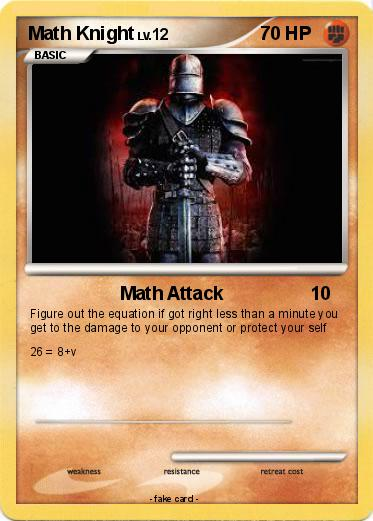Language Card : english

Creation Date : 17 March 2015

### Pokemon Passport

Name : Math Knight

Serie : Diamond & Pearl

Type : Fighting

Attack 1 : Math Attack
Figure out the equation if got right less than a minute you get to the damage to your opponent or protect your self 26 = 8+v

Attack 2 :

### Vote for this card

(1 vote)

Related cards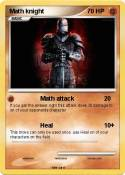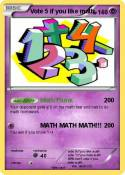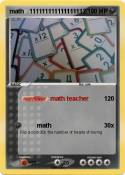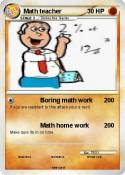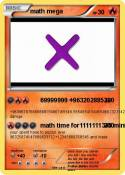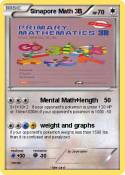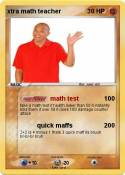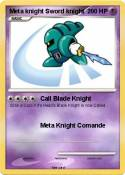JiJi ST math | The Great Math Wolf | Foxeh the math Fox | Khan academy | Vote 5 if you hate math | Math Mann | Ratey the Math Cat | I Can Do Math Everyday! | MATH | Math Teacher | MATh | math team | Math Book | Brice | Math | Math Teacher | Math :( | math 100 x | me in math | Math man | Daniel EX | Albert Einstein | MATH | knight guinto | Nerd | math | Math Homework | Cool Math | math doesn't lie | Tushar | Math 24 | Math Man | Math | Boring Math Teacher | Math | Mrs Ferriola | 1+1 | Ratey hte math cat | St Math | Mrs Baker | baldi | Math | Math | Math | Wall knight packs 999999 | knight cat | Sword Knight | Math | Math | more math | math | Asian guy | Asian guy | School | Math | prodigy guy | sonic the black knight | meta knight | Meta Knight | Dark Meta Knight | xtramath guy | Meta Knight | Meta Knight | Meta knight | Meta Knight | Knight Sonic | lord of the rings super knight | Meta knight | Galacta Knight 1,000,000,0 | Meta Knight